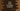# Java program to check if a number is perfect or not### Java program to find maximum and minimum values in a range of a list :

In this tutorial, we will learn how to find the maximum and minimum values in a list within range. Steps we are using in this Example program is as below :

### Steps to find maximum and minimum values in a list :

1. Get the total count of the numbers from the user.
2. Using a loop, get the inputs of the numbers. Add these numbers to a list.
3. Now, get the value of maximum and minimum of the range. We need to find the maximum and minimum of all numbers within this range.
4. Create two variables ’max’ and ’min’ to hold the maximum and minimum values .
5. Set values to both ’max’ and ’min’ as the first number of the list.
6. Iterate through the list one by one. If any number in the list lies in the maximum and minimum range, compare it with both ‘max’ and ‘min’.
7. If it is greater than ’max’, assign ’max’ to this number.
8. If it is smaller than ’min’, assign ’min’ to this number.
9. After the loop is completed, print out the ’max’ and ’min’ values.
10. Exit.

### Java Example :

``````import java.util.ArrayList;
import java.util.Scanner;

public class Main {

/**
* Utility function for System.out.println
*
* @param message : string to print
*/
private static void println(String message) {
System.out.println(message);
}

/**
* Utility function for System.out.print
*
* @param message : string to print
*/
private static void print(String message) {
System.out.print(message);
}

/**
* main method
*
* @throws java.lang.Exception
*/
public static void main(String[] args) throws java.lang.Exception {
Scanner scanner = new Scanner(System.in);
println("How many numbers you want to add to the list : ");

//read total count entered by the user
int totalCount = scanner.nextInt();

//create one arraylist to store the numbers
ArrayList numberList = new ArrayList();

//get the inputs from the user using a 'for' loop
for (int i = 0; i < totalCount; i++) {
print("Enter number " + (i + 1) + " : ");
int number = scanner.nextInt();

}

//minimum limit and maximum limit
int minLimit;
int maxLimit;

print("Enter minimum limit : ");
minLimit = scanner.nextInt();

print("Enter maximum limit : ");
maxLimit = scanner.nextInt();

int min = numberList.get(0);
int max = numberList.get(0);

//iterate through the list and find the max and min values
for (int i = 0; i < totalCount; i++) {
if (numberList.get(i) <= maxLimit && numberList.get(i) >= minLimit) {
if (numberList.get(i) > max) {
max = numberList.get(i);
}
if (numberList.get(i) < min) {
min = numberList.get(i);
}
}
}

//print the min and max values
println("Minimum value in the list : " + min);
println("Maximum value in the list : " + max);

}

}``````

### Sample Output :

``````How many numbers you want to add to the list :
7

Enter number 1 : 12
Enter number 2 : 23
Enter number 3 : 34
Enter number 4 : 56
Enter number 5 : 789
Enter number 6 : 761
Enter number 7 : 123
Enter minimum limit : 0
Enter maximum limit : 100
Minimum value in the list : 12
Maximum value in the list : 56``````